# Mathematical Expressions

## Superscripts and Subscripts

As in LaTeX, the carat (^) is used for superscripts and the underscore (_) is used for subscripts. In general, curly braces should be put around the raised or lowered expression.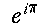e^{i\pi}

If the superscript or subscript consists only of a single character, the braces are not necessary.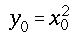y_0 = x_0^2

WebTeX can display tensor notation with the \tensor{}{} command. The first argument is the base, and the second argument contains a series of subscripts and superscripts marked with _ and ^. Pairs of ^ and _ are matched from left to right and aligned in the same column, regardless of order within the pair. If a ^ does not pair up with a _ when the pairs are made, then the missing subscript is left blank. In the example \tensor{R}{_i_k^j^m} below, the first subscript can not be paired up with a superscript, so the superscript's spot above the 'i' is blank. The same thing happens with the last superscript 'm'.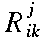\tensor{R}{_i^j_k}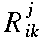\tensor{R}{^j_i_k}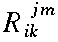\tensor{R}{_i_k^j^m}

The WebTeX Examples include more examples of superscripts and subscripts, as well as an example of tensor notation.

## Prescripts

Superscripts and subscripts may also be placed before an expression. These can be created with the \multiscripts{}{}{} command, a generalization of \tensor. Here, the second argument is the base, while the first argument contains the prescripts and the third argument contains the postscripts. These scripts are defined (by pairing up ^ and _) in the same way as they are for \tensor.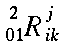\multiscripts{_0^2_1}{R}{_i^j_k}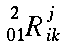\multiscripts{^2_0_1}{R}{^j_i_k}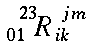\multiscripts{_0_1^2^3}{R}{_i_k^j^m}

The WebTeX Examples include an example of prescripts.

## Fractions and Binomial coefficients

Fractions may be created with \frac by placing the numerator in the first argument and the denominator in the second argument.

"One over x" is created with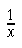\frac{1}{x}

The command \binom is similar to \frac. It places the first argument over the second argument, without drawing the horizontal fraction bar. To create a binomial coefficient, you will need to add parentheses with the \left ( and \right )commands. See the section on delimiters for further discussion of \left and \right.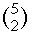\left ( \binom{5}{2} \right )

The WebTeX Examples include more examples of fractions.

## Square roots and n-th roots

The command \sqrt displays the square root of its argument.

For the n-th root, use the command \root with n as the first argument and the expression under the radical as the second argument.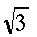\sqrt{3}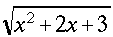\sqrt{x^2+2x+3}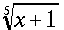\root{5}{x+1}

The WebTeX Examples include more examples of roots.

## Integrals, Sums, and other variable-sized operators

Some symbols will be larger in display style than they are in text style. These are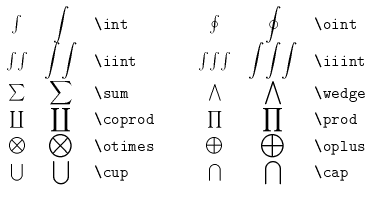Upper and lower limits for integrals or other variable size symbols can be specified with ^ and _ respectively. In display mode, these limits will be above and below the integral symbol. In text mode, the limits will be placed to the right of the symbol.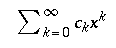\textstyle{ \sum_{k=0}^{\infty} c_k x^k }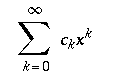\displaystyle{ \sum_{k=0}^{\infty} c_k x^k }
The symbol \iint draws a double integral, and \iiint draws a triple integral. These draw the integral symbols closer together than they normally would get with

\int \int or \int \int \int.

Use \displaystyle {\cup} to force the cup symbol to be large when the surrounding expressions are otherwise in text style.

The following five symbols will be large, regardless of the current style: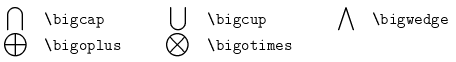The WebTeX Examples include examples of an integral in display style and variable-sized symbols.

## Logarithms and other functions

Many functions are traditionally typeset in an upright font. The following WebTeX commands automatically have this property.

\arccos   \cos   \csc   \exp   \ker     \limsup   \min   \sinh
\arcsin   \cosh  \deg   \gcd   \lg      \ln       \Pr    \sup
\arctan   \cot   \det   \hom   \lim     \log      \sec   \tan
\arg      \coth  \dim   \inf   \liminf  \max      \sin   \tanh


The limit functions will deal with subscripts and superscripts in a manner similar to the operators in the previous section. In display style, \lim_{x \to 0} f(x) will place the subscript underneath the limit symbol.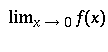\textstyle{\lim_{x \to 0} f(x)}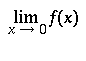\displaystyle{\lim_{x \to 0} f(x)}

If you have a function that is not on the list above, you may force any identifier to behave as an operator using the

\mathop{function}


command. For instance, the cosine function would normally be used this way:

\cos x


However, if the cosine function wasn't already on the list above, the command

\mathop{cos} x

would produce the same effect. That is, the "cos" would appear in an upright font, extra space would be included before the "x" and subscripts and superscripts would be treated as limits.

The WebTeX Examples include an example of log-like functions.

## Delimiters

Delimiters are the symbols such as parentheses and brackets which enclose a mathematical expression. These symbols, when used with the \left and \right commands, will grow to fit the size of the expression they enclose. The delimiters which WebTeX recognizes are: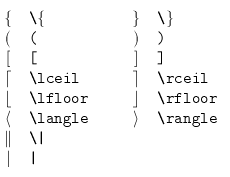Note that the curly braces must be specified with \{ and \} since braces alone are interpreted by WebEQ as part of the commands. A simple example is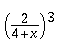\left ( \frac{2}{4+x} \right )^3

The \left and \right commands must be used in pairs, since WebTeX expects the expression that determines the height of the delimiters to be surrounded by a pair of \left and \right commands. However, only the \left and \right keywords must appear in pairs; either delimiter after the keyword may be omitted: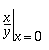\left \frac{x}{y} \right|_{x=0}

Caution: This is a significant difference between WebTeX and TeX. In TeX, some delimiter is required after the \right or \left command and uses a special invisible delimiter in situations like the one illustrated above.

By default, delimiters grow symmetrically around the horizontal axis of the equation. When enclosing a matrix that is aligned at its top or bottom, for example, one needs the delimiters to "float" to match the alignment of the enclosed expression. To accomplish this, use the \floatleft and \floatright commands instead. For matching purposes, a \floatleft will match a plain \right and vice versa.

The WebTeX Examples include more examples of delimiters.

## Accents

There are seven accents which place a one-character wide accent above their arguments: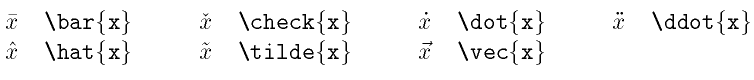The wide versions of these five accents stretch to the width of the enclosed expression.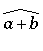\widehat{a+b}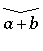\widecheck{a+b}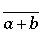\widebar{a+b}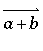\widevec{a+b}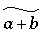\widetilde{a+b}

The WebTeX Examples include more examples of accents.

## Placing symbols over or under an expression

The command \overbrace places a horizontal brace that stretches over its argument, and \underbrace stretches a brace underneath its argument.

Besides braces, any symbol may be placed above or below an expression with the commands \overset or \underset. These are generalizations of \overbrace and \underbrace. Instead of a brace, any symbols that are in the first argument will be typeset above or below the main expression in the second argument.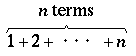\overset{\text{$n$ terms}}{\overbrace{1+2+\cdots+n}}

The WebTeX Examples include more examples of the overbrace and overset commands.

## Mathematical symbols and extended characters

There are many other mathematical symbols which are supported by WebTeX.

Both capital and lower-case Greek letters are available. The capital letters that are not listed on the table below are the same as the Roman capitals.

Capital letters: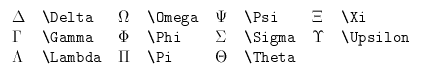Lower-case letters: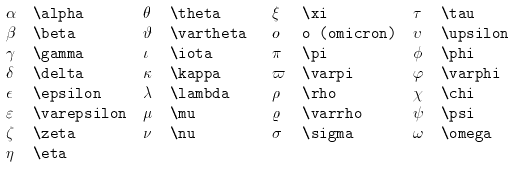Arrows: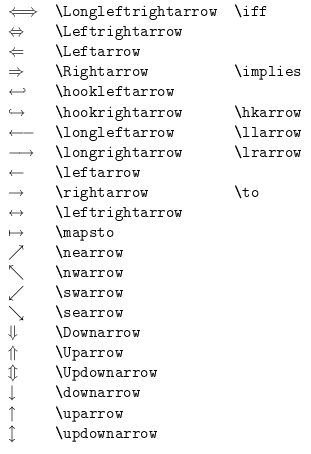Relations: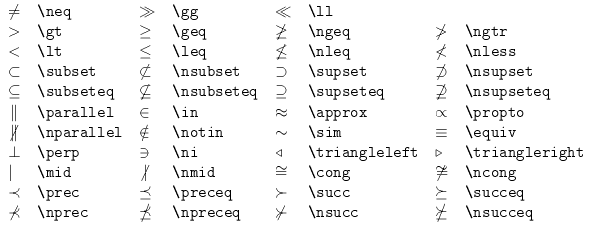Binary operations: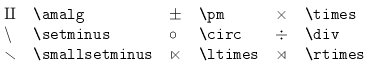Dots: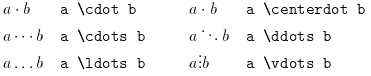Miscellaneous Symbols: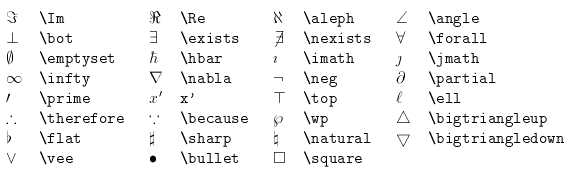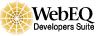Created: Dec 18 1997 --- Last modified: Tue Oct 23 17:24:20 2001